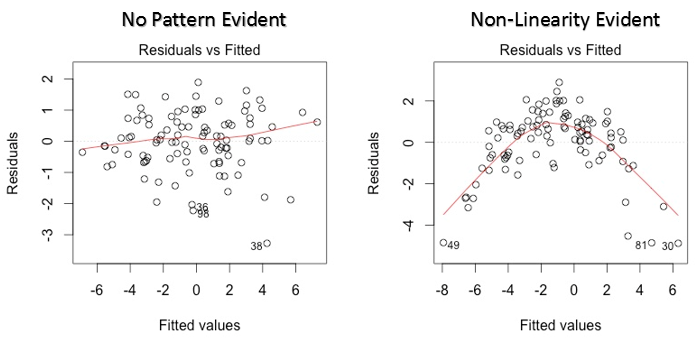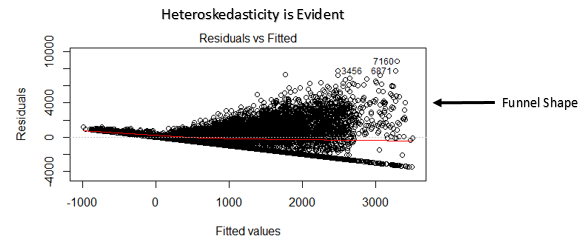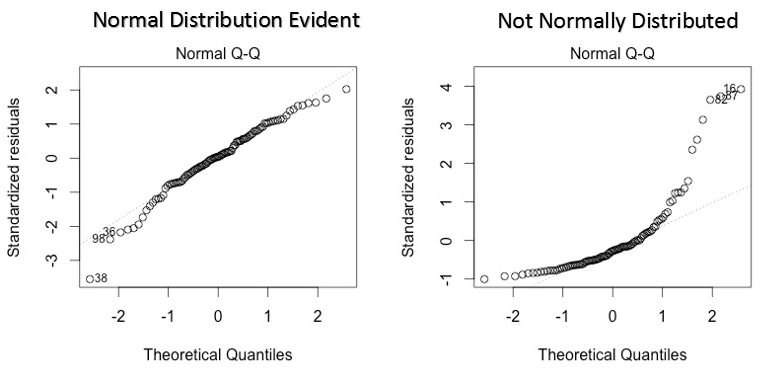Analytics Vidhya — July 14, 2016

## Introduction

All models are wrong, but some are useful – George Box

Regression analysis marks the first step in predictive modeling. No doubt, it’s fairly easy to implement. Neither it’s syntax nor its parameters create any kind of confusion. But, merely running just one line of code, doesn’t solve the purpose. Neither just looking at R² or MSE values. Regression tells much more than that!

In R, regression analysis return 4 plots using `plot(model_name)` function. Each of the plot provides significant information or rather an interesting story about the data. Sadly, many of the beginners either fail to decipher the information or don’t care about what these plots say. Once you understand these plots, you’d be able to bring significant improvement in your regression model.

For model improvement, you also need to understand regression assumptions and ways to fix them when they get violated.

In this article, I’ve explained the important regression assumptions and plots (with fixes and solutions) to help you understand the regression concept in further detail. As said above, with this knowledge you can bring drastic improvements in your models.

Note: To understand these plots, you must know basics of regression analysis. If you are completely new to it, you can start here. Then, proceed with this article.## Assumptions in Regression

Regression is a parametric approach. ‘Parametric’ means it makes assumptions about data for the purpose of analysis. Due to its parametric side, regression is restrictive in nature. It fails to deliver good results with data sets which doesn’t fulfill its assumptions. Therefore, for a successful regression analysis, it’s essential to validate these assumptions.

So, how would you check (validate) if a data set follows all regression assumptions? You check it using the regression plots (explained below) along with some statistical test.

Let’s look at the important assumptions in regression analysis:

1. There should be a linear and additive relationship between dependent (response) variable and independent (predictor) variable(s). A linear relationship suggests that a change in response Y due to one unit change in X¹ is constant, regardless of the value of X¹. An additive relationship suggests that the effect of X¹ on Y is independent of other variables.
2. There should be no correlation between the residual (error) terms. Absence of this phenomenon is known as Autocorrelation.
3. The independent variables should not be correlated. Absence of this phenomenon is known as multicollinearity.
4. The error terms must have constant variance. This phenomenon is known as homoskedasticity. The presence of non-constant variance is referred to heteroskedasticity.
5.  The error terms must be normally distributed.

## What if these assumptions get violated ?

Let’s dive into specific assumptions and learn about their outcomes (if violated):

1. Linear and Additive:  If you fit a linear model to a non-linear, non-additive data set, the regression algorithm would fail to capture the trend mathematically, thus resulting in an inefficient model. Also, this will result in erroneous predictions on an unseen data set.

How to check: Look for residual vs fitted value plots (explained below). Also, you can include polynomial terms (X, X², X³) in your model to capture the non-linear effect.

2. Autocorrelation: The presence of correlation in error terms drastically reduces model’s accuracy. This usually occurs in time series models where the next instant is dependent on previous instant. If the error terms are correlated, the estimated standard errors tend to underestimate the true standard error.

If this happens, it causes confidence intervals and prediction intervals to be narrower. Narrower confidence interval means that a 95% confidence interval would have lesser probability than 0.95 that it would contain the actual value of coefficients. Let’s understand narrow prediction intervals with an example:

For example, the least square coefficient of X¹ is 15.02 and its standard error is 2.08 (without autocorrelation). But in presence of autocorrelation, the standard error reduces to 1.20. As a result, the prediction interval narrows down to (13.82, 16.22) from (12.94, 17.10).

Also, lower standard errors would cause the associated p-values to be lower than actual. This will make us incorrectly conclude a parameter to be statistically significant.

How to check: Look for Durbin – Watson (DW) statistic. It must lie between 0 and 4. If DW = 2, implies no autocorrelation, 0 < DW < 2 implies positive autocorrelation while 2 < DW < 4 indicates negative autocorrelation. Also, you can see residual vs time plot and look for the seasonal or correlated pattern in residual values.

3. Multicollinearity: This phenomenon exists when the independent variables are found to be moderately or highly correlated. In a model with correlated variables, it becomes a tough task to figure out the true relationship of a predictors with response variable. In other words, it becomes difficult to find out which variable is actually contributing to predict the response variable.

Another point, with presence of correlated predictors, the standard errors tend to increase. And, with large standard errors, the confidence interval becomes wider leading to less precise estimates of slope parameters.

Also, when predictors are correlated, the estimated regression coefficient of a correlated variable depends on which other predictors are available in the model. If this happens, you’ll end up with an incorrect conclusion that a variable strongly / weakly affects target variable. Since, even if you drop one correlated variable from the model, its estimated regression coefficients would change. That’s not good!

How to check: You can use scatter plot to visualize correlation effect among variables. Also, you can also use VIF factor. VIF value <= 4 suggests no multicollinearity whereas a value of >= 10 implies serious multicollinearity. Above all, a correlation table should also solve the purpose.

4. Heteroskedasticity: The presence of non-constant variance in the error terms results in heteroskedasticity. Generally, non-constant variance arises in presence of outliers or extreme leverage values. Look like, these values get too much weight, thereby disproportionately influences the model’s performance. When this phenomenon occurs, the confidence interval for out of sample prediction tends to be unrealistically wide or narrow.

How to check: You can look at residual vs fitted values plot. If heteroskedasticity exists, the plot would exhibit a funnel shape pattern (shown in next section). Also, you can use Breusch-Pagan / Cook – Weisberg test or White general test to detect this phenomenon.

5. Normal Distribution of error terms: If the error terms are non- normally distributed, confidence intervals may become too wide or narrow. Once confidence interval becomes unstable, it leads to difficulty in estimating coefficients based on minimization of least squares. Presence of non – normal distribution suggests that there are a few unusual data points which must be studied closely to make a better model.

How to check: You can look at QQ plot (shown below). You can also perform statistical tests of normality such as Kolmogorov-Smirnov test, Shapiro-Wilk test.

## Interpretation of Regression Plots

Until here, we’ve learnt about the important regression assumptions and the methods to undertake, if those assumptions get violated.

But that’s not the end. Now, you should know the solutions also to tackle the violation of these assumptions. In this section, I’ve explained the 4 regression plots along with the methods to overcome limitations on assumptions.

1. Residual vs Fitted ValuesThis scatter plot shows the distribution of residuals (errors) vs fitted values (predicted values). It is one of the most important plot which everyone must learn. It reveals various useful insights including outliers. The outliers in this plot are labeled by their observation number which make them easy to detect.

There are two major things which you should learn:

1. If there exist any pattern (may be, a parabolic shape) in this plot, consider it as signs of non-linearity in the data. It means that the model doesn’t capture non-linear effects.
2. If a funnel shape is evident in the plot, consider it as the signs of non constant variance i.e. heteroskedasticity.

Solution: To overcome the issue of non-linearity, you can do a non linear transformation of predictors such as log (X), √X or X² transform the dependent variable. To overcome heteroskedasticity, a possible way is to transform the response variable such as log(Y) or √Y. Also, you can use weighted least square method to tackle heteroskedasticity.

2. Normal Q-Q PlotThis q-q or quantile-quantile is a scatter plot which helps us validate the assumption of normal distribution in a data set. Using this plot we can infer if the data comes from a normal distribution. If yes, the plot would show fairly straight line. Absence of normality in the errors can be seen with deviation in the straight line.

If you are wondering what is a ‘quantile’, here’s a simple definition: Think of quantiles as points in your data below which a certain proportion of data falls. Quantile is often referred to as percentiles. For example: when we say the value of 50th percentile is 120, it means half of the data lies below 120.

Solution: If the errors are not normally distributed, non – linear transformation of the variables (response or predictors) can bring improvement in the model.

3. Scale Location PlotThis plot is also used to detect homoskedasticity (assumption of equal variance). It shows how the residual are spread along the range of predictors. It’s similar to residual vs fitted value plot except it uses standardized residual values. Ideally, there should be no discernible pattern in the plot. This would imply that errors are normally distributed. But, in case, if the plot shows any discernible pattern (probably a funnel shape), it would imply non-normal distribution of errors.

Solution: Follow the solution for heteroskedasticity given in plot 1.

4. Residuals vs Leverage PlotIt is also known as Cook’s Distance plot. Cook’s distance attempts to identify the points which have more influence than other points. Such influential points tends to have a sizable impact of the regression line. In other words, adding or removing such points from the model can completely change the model statistics.

But, can these influential observations be treated as outliers? This question can only be answered after looking at the data. Therefore, in this plot, the large values marked by cook’s distance might require further investigation.

Solution: For influential observations which are nothing but outliers, if not many, you can remove those rows. Alternatively, you can scale down the outlier observation with maximum value in data or else treat those values as missing values.

## End Notes

You can leverage the true power of regression analysis by applying the solutions described above. Implementing these fixes in R is fairly easy. If you want to know about any specific fix in R, you can drop a comment, I’d be happy to help you with answers.

My motive of this article was to help you gain the underlying knowledge and insights of regression assumptions and plots. This way, you would have more control on your analysis and would be able to modify the analysis as per your requirement.

Did you find this article useful ? Have you used these fixes in improving model’s performance? Share your experience / suggestions in the comments.

### You can test your skills and knowledge. Check out Live Competitions and compete with best Data Scientists from all over the world.###### Analytics Vidhya

This is the official account of the Analytics Vidhya team.

## 23 thoughts on "Going Deeper into Regression Analysis with Assumptions, Plots & Solutions"###### Aarshay Jain says:July 14, 2016 at 6:51 am
Thanks for the really nice article. this part of regression is mostly missed by many. Reply###### Analytics Vidhya Content Team says:July 14, 2016 at 6:55 am
Glad you found it helpful. I've seen regression algorithm shows drastic model improvements when used with techniques I described above. I hope it help others as well. Reply###### Vivek Gaur says:July 15, 2016 at 2:10 am
Small edit: Durbin Watson d values always lie between 0 and 4. A value of d=2 indicates no autocorrelation. A value between 0-2 indicates positive correlation while a value between 2-4 indicates negative correlation. Please correct the blog. Reply###### Rahul Sangole says:July 15, 2016 at 2:29 am
This is a good article. I have a comment on the Residuals vs Leverage Plot and the comment about it being a Cook's distance plot. Although you mention this as a Cook's distance plot, and mark Cook's distance at std residual of -2, this seems incorrect. It looks like you have plotted standardized residuals e=(I-H)y vs leverage (hii from hat matrix H). And the +/- 2 cutoff is typically from R-student residuals. If you had plotted Cook's distance, the cutoff would typically be 1 or 4/n. Correct me if I'm wrong here, thanks! Reply###### Analytics Vidhya Content Team says:July 15, 2016 at 4:07 am
Thanks Vivek. I just checked and found that's correct. Made the changes. Reply###### Analytics Vidhya Content Team says:July 15, 2016 at 4:59 am
Hi Rahul, I think the marked cook's distance at -2 is just a legend which shows cook's distance can be determined by the red dotted line. After creating, residual vs leverage plots based on other data sets, I came to this conclusion. Reply###### john says:July 15, 2016 at 6:56 am
I find the article useful especially for guys planning to join data analytic field. However, I find this number two and three confusing There should be no correlation between the residual (error) terms. Absence of this phenomenon is known as Autocorrelation. The independent variables should not be correlated. Absence of this phenomenon is known as multicollinearity. Reply###### tom reilly says:July 15, 2016 at 4:44 pm
The stages of modeling are Identification, Estimation,Diagnostic checking and then Forecasting as laid out by Box-Jenkins in their 1970 text book "Time Series Analysis: Forecasting and Control". The idea is to identify if there is relationship using the cross-correlation function instead of assuming one. In fact, there might be MSB (model specification bias)if you assume. There might be a lead or lag relationship to complicate matters. A bivariate normalized scatter plot is also very helpful. The one item that no one ever covers (except us) is looking for outliers and changes with multivariate data(change in trend, level, seasonality,parameters,variance). If you aren't looking for these then you just skipped "diagnostic checking" so that your residuals are random with constant mean and variance. Try this example and see how you do....http://bit.ly/29kLC1g Good luck! Reply###### akrsrivastava says:July 15, 2016 at 5:47 pm
Thanks for the writeup.. How can we identify which predictors from a large set of predictors have a non-linear impact on the model? Reply###### urna kundu says:July 15, 2016 at 7:24 pm
Regarding the first assumption of regression;"Linearity"-the linearity in this assumption mainly points the model to be linear in terms of parameters instead of being linear in variables and considering the former, if the independent variables are in the form X^2,log(X) or X^3;this in no way violates the linearity assumption of the model. Then how can I use these polynomial terms to correct non linearity, when there presence, with linear parametrs is maintaining the model's linearity assumption. Reply###### JOSE LUIS CRUZ says:July 15, 2016 at 10:53 pm
All your contributions are very useful for professionals and non professionals. I appreciate your availability to share the must know issues to get better society. Reply###### Rahul Sangole says:July 16, 2016 at 12:27 am
Manish, you must pick one or the other. Cook's Distance is not Leverage. Cook's Distance is a function of studentized residuals, and the diagonals of the hat matrix; on the other hand, leverage is simply the diagonal elements of the hat matrix. Reply###### urna kundu says:July 16, 2016 at 3:31 am
when considering the linearity assumption, are you considering the model to be linear in variables only or linear in parameters only? Reply###### Ramit Pandey says:July 16, 2016 at 5:49 am
Hi Manish, Can You Plz suggest the the best book to study Data analysis so deep as you explained in your article. I want to study root concepts so that my thinking ability enhances . Thanks a million. Ramit Pandey Reply###### Analytics Vidhya Content Team says:July 16, 2016 at 6:39 am
Hi Ramit, If you want to learn from scratch, you can read Introduction to Statistical Learning. It's freely available in PDF version. Reply###### Ankur Bhargava says:July 16, 2016 at 7:47 pm
Hi Manish , Could you please explain the scaling of these graphs ? what does -2 , -4 , 4 , 6, 8 represent on X axis and Y axis ? Thanks, Ankur Reply###### Jack says:July 21, 2016 at 2:54 pm
Really good article. Thank you so much. Could you please give us some explanation about logistic regression with these plots? Reply###### Allen says:August 03, 2016 at 5:49 pm
Same with Jack. Could you please share an article about Logistic Regression analysis? Thank you so much!!! Reply###### Akhil Das says:August 06, 2016 at 4:33 pm
I would like to differ on the assumptions of linear regression. Linear regression does not make any direct assumption about the zero auto-correlation of the error terms. It does assume that the observations are randomly selected after the assumption of linearity and additive phenomena. Also the last assumption of normality of error terms is relaxed when a sufficiently large sample of data is present. Reply###### Akshat says:August 06, 2016 at 8:41 pm
Awesome job mate with the plots, assumptions and explanation ... A few tests for normality i never heard of .... Please do one for logistic regression also XD Reply###### nba 2k17 coins says:August 11, 2016 at 8:35 am
Wow cuz this is very helpfulexcellent work! Congrats and keep it up Reply###### Ramsey says:October 16, 2016 at 6:38 pm
It really helps when these topics are broken down in an intuitive and consumable way. And you did it very well! Can't wait to read more... Reply###### Pratik Solanki says:November 12, 2016 at 4:36 am
Can you explain heteroskedasticiy more in detail .I am not able to understand it properly.Is it always the funnel which defines heteroskedasticiy in the model. Reply